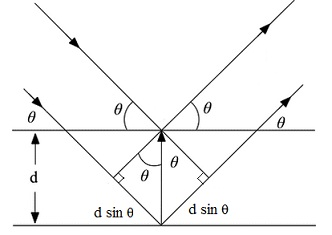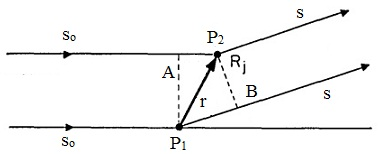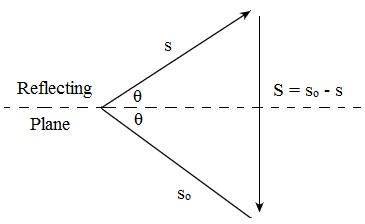#### Crystal Diffraction-I, Physics tutorial

Introduction:

In order to look at the structure of crystals we need waves that interact by atoms and which encompass a wavelength comparable by inter atomic spacing in crystals; that is, we need a wavelength of the order of 1 A (= 10-8 cm). The interaction must be weak adequate in such a way that the wave can penetrate in a coherent fashion into the crystal for a distance of the order of perhaps 1000 lattice constants. The most convenient waves appropriate for our purpose are those related by the x-rays, whereas the waves related by the neutrons and electrons have found vital special applications. Whenever an atom is exposed to the electromagnetic radiation, the atomic electrons are accelerated, and they radiate at the frequency of the incident radiation. The superposition of the waves scattered through individual atoms in a crystal outcomes in the ordinary optical refraction. Whenever the wavelength of the radiation is comparable by or smaller than the lattice constant, we will as well under some conditions have diffraction of the incident beam.

Definition:

If a monochromatic beam of x-rays is shone on a regular crystalline material then the beam will be scattered from the material at definite angles. This generated an interference effect termed as diffraction between the X-rays from various layers within the crystal.

Bragg formulation of diffraction by a crystal:

W. L. Bragg in the year 1913 discovered that one could account for the position of the diffracted beams generated by a crystal in an x-ray beam through a very simple model according to which x-rays are reflected from different planes of atoms in the crystal. The diffracted beams are found for case in which the reflections from parallel planes of atoms interfere constructively. The derivation of the Bragg law is represented in the figure shown below. We assume that in the crystal a sequence of atomic planes that are partly reflecting for radiation of wavelength X and which spaced equivalent distances d are apart. The radiation is incident in the plane of the paper. The path difference for rays reflected from the adjacent planes is 2 d sin θ. Reinforcement of the radiation reflected from the successive planes will take place if the path difference is an integral number 'n' of wavelengths. The condition for constructive reflection is:

2 d sin θ = nλ

The above equation represents the Bragg law. The integer 'n' represents the order of corresponding reflection. This must be emphasized that the Bragg equation results from the periodicity of the structure, devoid of reference to the composition of the unit of repetition.Fig: Derivation of the Bragg equation 2 d sin θ = nλ, here 'd' is the spacing of the parallel atomic planes

Statement of Bragg's law with two geometrical facts:

Bragg's law of diffraction defines that the path difference between the two X-rays which are reflected from the adjacent planes is an integral multiple of the wavelength, that is,

2 d sin θ = nλ

Here,

θ = Bragg's angle

d = Interatomic plane spacing

λ = Wavelength of the X-rays

n = order of diffraction

The two geometrical facts are:

1) The incident beam, the normal to the diffraction plane and the diffracted beam are for all time coplanar.

2) The angle between the diffracted beams is for all time 2θ. This is termed as the diffraction angle.

Von Laue formulation of diffraction by a crystal:

Assuming the nature of x-ray diffraction pattern generated by similar atoms positioned at the corners (that is, lattice points) of the primitive cells of a space lattice to examine the scattering from any two lattice points, P1 and P2 (as shown in figure below) separated by the vector 'r'. The unit incident wave normal is 'so' and the unit scattered wave normal is 's'. Let us examine at a point a long distance away the difference in phase of the radiation scattered through P1 and P2. If P1B and P2A are the projections of 'r' on the incident and scattered wave directions, then the path difference among the two scattered waves is:

P1B - P2A = r.s - r.so = r . (s - so)Fig: Computation of the Phase difference of the waves scattered from the two lattice points

The vector s - so = S consists of a simple interpretation (as shown in figure) as the direction of the normal to a plane that would reflect the incident direction to the scattering direction. This plane is a helpful mathematical construction and this is termed as the reflecting plane. If '2θ' is the angle 's' makes with so, then 'θ' is the angle of incidence, and from the figure, we observe that |S| = 2 sin θ, as 's' and 'so' are the unit vectors.The phase difference Φ = 2π/λ times the path difference. We have:

Φ = 2π/λ (r.s)

The amplitude of the scattered wave is maximum in a direction such that the contributions from each and every lattice point differ in phase only by integral multiplies of 2π. This is satisfied when the phase difference among the two adjacent lattice points is an integral multiple of 2π. If a, b, c are the basis vectors, we should have for the diffraction maxima:

Φa = 2π/λ (a.s) = 2πh;

Φb = 2π/λ (b.s) = 2πk;

Φc = 2π/λ (c.s) = 2πl;

Here h, k, l are the integers

If α, β, γ are the direction cosines of S with respect to a, b, c, then we have:

a.s = 2aα sinθ = hλ

b.s = 2aβ sinθ = kλ

c.s = 2aγ sinθ = lλ

The above two set of equations are the Laue equations. They have solutions only for special values of θ and the Wavelength λ. The Laue equations have a simple geometrical interpretation. The Laue equations define that in a diffraction direction the direction cosines are proportional to the h/a, k/b, l/c, correspondingly and the adjacent lattice planes (hkl) intersect the axes at intervals a/h, b/k, c/l in such a way that via elementary plane geometry the direction cosines of the normal to (hkl) are proportional to h/a, k/b, l/c correspondingly. Thus the lattice planes (hkl) should be parallel to the reflecting plane. If d (hkl) is the spacing among the two adjacent planes of a set (hkl), we have by projection:

d (hkl) = aα/h = bβ/k = cγ/l

Then, from the equation we have:

2d (hkl) sin θ = λ

We might interpret by giving an extended meaning to the spacing d (hkl) when h, k, l encompass a common factor n: the diffracted wave in reality occurs from the nth order reflection from the true lattice planes, however we might as a mathematical device think of the diffracted wave as a first order reflection from the set of planes parallel to the true lattice planes however with a spacing d (hkl) equivalent to l/n of the true spacing.

Diffraction of crystals by electrons:

De Broglie in the year 1924 predicted that the wavelength related by a particle of momentum p = mv is represented by:

λ = h/p

Here 'h' is plank's constant. One of the most direct pieces of proof of the wave aspect of particles was given by the electron diffraction experiments of Davisson and Germer in the year 1972. They concluded that if one associates a wavelength by the electrons given by above equation, the diffraction pattern acquired can be interpreted in precisely the same way as the X-ray diffraction patterns. As long as the velocity of the electrons is small compared by the velocity of light, the wavelength of the electrons might be deduced in terms of the accelerating voltage V as follows

1/2 mv2 = eV or λ = h/(2meV)1/2 ≈ (150/V)1/2

'λ' is obtained in Angstroms if 'V' is represented in volts. Note that only 150 volts are needed to generate electrons of a wavelength of 1Ao compared with X-rays that require around 12,000 volts for 1Ao. Electrons are scattered through the nucleus and also by the electrons in the atoms. For spherical charge distribution one can exhibit that the scattering factor is represented by:

E(θ) = (me2/2h2) (Z - fs) (λ2/sin2 θ)

In this, 'fs' is the scattering factor for X-rays, 'Z' is the nuclear charge and 'θ' is the Bragg angle. As for X-rays the scattering factor reduces by increasing the values of θ. Though, there is a considerable difference between X-rays and electrons in that, electrons are scattered much more efficiently through atoms than are X-rays. However, atoms scattered electrons more strongly by some powers of ten for the energy involved. At normal incidence an electron of around 50 keV consists of a penetration depth for elastic scattering of only around 500 Ao, while for the small angles of incidence used in reflection methods this might be around 50 k measured perpendicularly to the surface. It is evident; thus, that electron diffraction is specifically helpful in investigating the structure of thin surface layers like oxide on metals. These layers would not be detected through X-rays diffraction as the patterns acquired are features for the bulk material.

Diffraction of crystals by neutrons:

The mass of a neutron is approximately 2000 as large as that of an electron, in such a way that according to equation λ = h/p the wavelength related with a neutron is around 1/2000 that for an electron of the similar velocity. Therefore the energy of a neutron needed to give 1 Ao is of order of only 0.1eV. Such neutrons can be achieved from a chain-reacting pile, and diffraction from crystals might be observed. Neutrons are scattered basically by the nuclei of the atoms, apart from when they are magnetic. The radius of atomic nuclei is of the order of 10-13cm, and as a consequence, the atomic scattering factor is almost independent of the scattering angle, as λ >> 10-13 cm. As well, the scattering power doesn't differ in a regular way by the atomic number, in such a way that light elements like hydrogen and carbon still generate relatively strong scattering.  The scattering of X-rays through light element is in contrast, of course, relatively weak. Therefore the positions of such atoms in crystalline solids might be determined from the neutron diffraction experiments. The other significant feature of neutron diffraction is the fact that scattering from neighboring elements in the periodic system might differ appreciably. For illustration neutron diffraction permits one to detect with relative ease ordered phases of an alloy such as FeCo, while their detection by X-rays is difficult. A particularly significant feature of neutron diffraction is their use in investigating the magnetic structure of solids. This is an outcome of the interaction between the magnetic moment of the neutron and that of the atoms concerned. In the paramagnetic substance, in which the magnetic moments are arbitrarily oriented in space, this leads to the incoherent scattering, resultant in a diffuse background. This diffuse background of magnetic scattering is then superimposed on the lines generated by the nuclear scattering illustrated above. In a ferromagnetic substance in which the magnetic moments in a domain are lined up in parallel, this diffuse background is absent. In an antiferromagnetic solid, the magnetic moments of specific pairs of atoms are aligned antiparallel and therefore, from the view-point of the neutron, such atoms would appear to be dissimilar.

Tutorsglobe: A way to secure high grade in your curriculum (Online Tutoring)